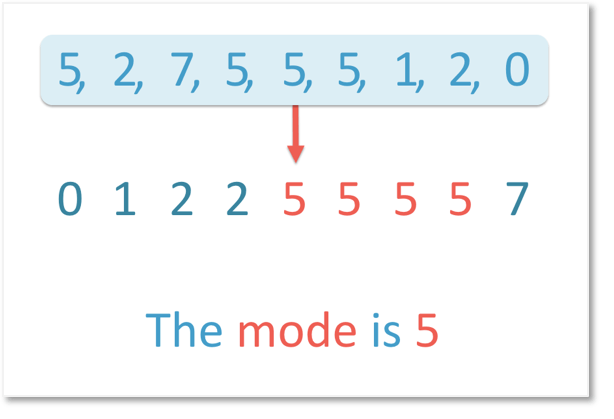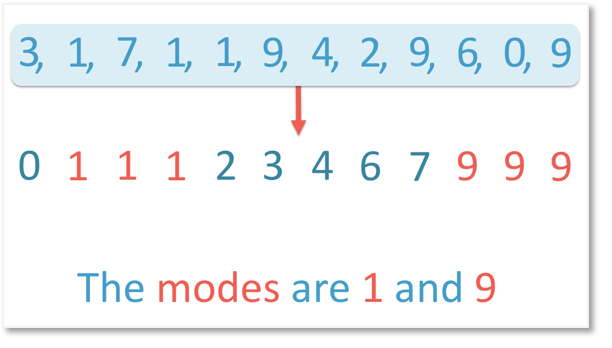# Finding the Mode

How to Find the Mode• This set of numbers is: 5, 2, 7, 5, 5, 5, 1, 2, 0.
• The mode is the number that appears the most.
• It is easier to see the mode by putting the numbers in order from smallest to largest.
• In order, the numbers are: 0, 1, 2, 2, 5, 5, 5, 5, 7.
• The most frequent number is 5.
• There are four number fives.
• 5 is the mode.

The mode is the most frequent number in a set of data.

The mode is the number that appears the most.• We will find the mode of the set of numbers: 3, 1, 7, 1, 1, 9, 4, 2, 9, 6, 0, 9.
• We begin by writing the numbers in order from smallest to largest.
• We have: 0, 1, 1, 1, 2, 3, 4, 6, 7, 9, 9, 9.
• There are three 1’s and also three 9’s.
• The most common number is both 1 and 9.
• We say that the mode is 1 and 9. We have two modes in this example.
• Data that has two modes is called bimodal.Supporting Lessons# How to Find the Mode of a Set of Numbers

The mode is the most frequent value in a data set. It is the number that appears the most.

To find the mode of a set of numbers use the following steps:

1. Count how many of each number there are in total.
2. The mode is the number that appears the most.
3. If there is more than one of these numbers, then they are all the mode.
4. If all of the numbers appear in equal amounts then there is no mode.

When there are only a small amount of numbers in the list, it can be easiest to count the numbers in the list immediately.

However, for larger lists of numbers, we can first write the list of numbers in order from smallest to largest. This can help us to count how many of each number there are.

Here is an example of finding the mode from a list of numbers.

We have: 5, 2, 7, 5, 5, 5, 1, 2, 0.

We can write the numbers in order as:

0, 1, 2, 2, 5, 5, 5, 5, 7.

When rewriting the numbers in order, you need to make sure that you have written all of the numbers once. It can help to cross numbers off the original list as you rewrite them.

You should also count how many numbers there were to begin with and count how many are in your rewritten list.

Here there were 9 numbers in total and in the rewritten list, we have 9 numbers.From the list of: 0, 1, 2, 2, 5, 5, 5, 5, 7 we can count the amount of each number.

We can see that there are two 2’s and four 5’s.

5 is the number that appears the most and so, 5 is the mode.In this example, we have a large list of numbers.

We have 12 numbers: 3, 1, 7, 1, 1, 9, 4, 2, 9, 6, 0, 9.

To find the most of this list of numbers, we first write the numbers in order.

In order from smallest to the largest, the numbers are:

0, 1, 1, 1, 2, 3, 4, 6, 7, 9, 9, 9.

We can count these numbers to check that we wrote all 12 numbers.In this example, the most common numbers are both 1 and 9.

There are three 1’s and three 9’s.

We say that the data is bimodal. Bimodal means that there are exactly two modes.

If the data is bimodal we write them both as our answer. We do not average the modes.This data was bimodal so we wrote both 1 and 9 as our mode.

If there are many modes, then we can call the data multimodal.

If every single number appears the exact same amount of times, then we say the data does not have a mode.Now try our lesson on How to Find the Range where we learn what the range of a set of numbers is.